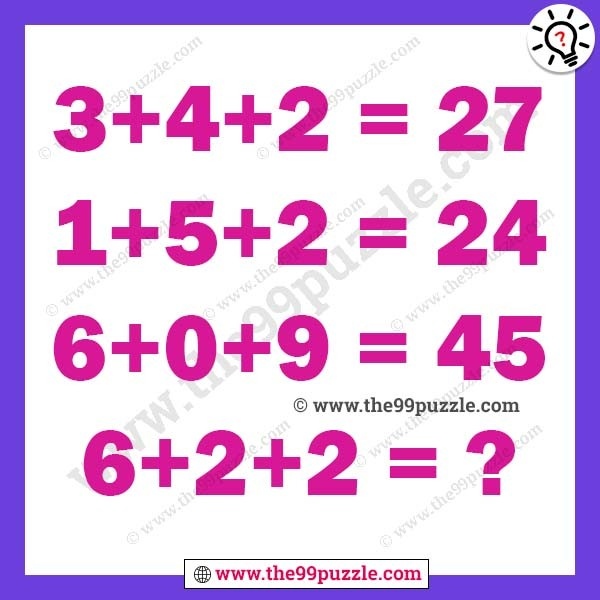# Solve the logical reasoning math puzzle with answer – Puzz202

Here this logical reasoning math puzzle picture you get some math equations. All these equations are logical. Can you find the math logic and find out the answer. When you try to solve this type of puzzle, think logically and mathematically. A simple trick applies and finds out the last equation value.

3+4+2=27

1+5+2=24

6+0+9=45

6+2+2=?###### Explanation:

3+4+2=(3+4+2)×3=27

1+5+2=(1+5+2)×3=24

6+0+9=(6+0+9)×3=45

6+2+2=(6+2+2)×3=30